# coloring

## Crossing numbers and coloring ★★★

Author(s): Albertson

We letdenote the crossing number of a graph.

Conjecture   Every graphwithsatisfies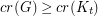.

Keywords: coloring; complete graph; crossing number

## Are vertex minor closed classes chi-bounded? ★★

Author(s): Geelen

Question   Is every proper vertex-minor closed class of graphs chi-bounded?

Keywords: chi-bounded; circle graph; coloring; vertex minor

## Graphs with a forbidden induced tree are chi-bounded ★★★

Author(s): Gyarfas

Say that a familyof graphs is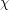-bounded if there exists a function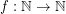so that everysatisfies.

Conjecture   For every fixed tree, the family of graphs with no induced subgraph isomorphic tois-bounded.

Keywords: chi-bounded; coloring; excluded subgraph; tree

## Domination in plane triangulations ★★

Author(s): Matheson; Tarjan

Conjecture   Every sufficiently large plane triangulationhas a dominating set of size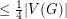.

Keywords: coloring; domination; multigrid; planar graph; triangulation

## Double-critical graph conjecture ★★

Author(s): Erdos; Lovasz

A connected simple graphis called double-critical, if removing any pair of adjacent vertexes lowers the chromatic number by two.

Conjecture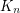is the only-chromatic double-critical graph

Keywords: coloring; complete graph

## Counting 3-colorings of the hex lattice ★★

Author(s): Thomassen

Problem   Find.

Keywords: coloring; Lieb's Ice Constant; tiling; torus

## 4-regular 4-chromatic graphs of high girth ★★

Author(s): Grunbaum

Problem   Do there exist 4-regular 4-chromatic graphs of arbitrarily high girth?

Keywords: coloring; girth

## Coloring random subgraphs ★★

Author(s): Bukh

Ifis a graph and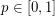, we letdenote a subgraph ofwhere each edge ofappears inwith independently with probability.

Problem   Does there exist a constantso that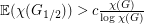?

Keywords: coloring; random graph

## Hedetniemi's Conjecture ★★★

Author(s): Hedetniemi

Conjecture   If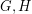are simple finite graphs, then.

Here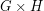is the tensor product (also called the direct or categorical product) ofand.

## Degenerate colorings of planar graphs ★★★

Author(s): Borodin

A graphis-degenerate if every subgraph ofhas a vertex of degree.

Conjecture   Every simple planar graph has a 5-coloring so that for, the union of anycolor classes induces a-degenerate graph.

Keywords: coloring; degenerate; planar